### Transient Vibrations: Convolution

The method of convolution is based upon the response to an impulse load. We have shown previously in equation (7.4) that the response to an impulse applied at time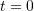is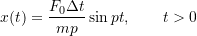where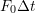is the magnitude of the impulse. To proceed, we next need to consider the response of the system due to an impulse that is applied at some other time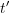.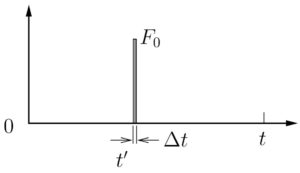Figure 7.8: Impulse applied at timeWe are interested in the response of the mass at some later time, where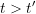. In such a case the response of the mass at timedue to an impulse applied at an earlier timeis simply

(7.9)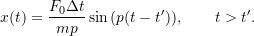where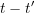is the elapsed time after the impulse was applied. Note that this assumes that the mass is at rest at time(ie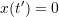and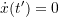). Equation (7.9) then gives us the position of the mass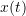at time, which is after the impulse has been applied. Note that with the assumed initial conditions, the response of the mass if the impulse were not applied would be simply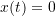. Therefore, what (7.9) in fact represents is the change in the response, compared to what would have occurred if the impulse had not been applied.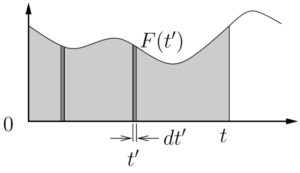Figure 7.9: Forcing function represented as a series of impulses

Finally, consider some arbitrary forcing function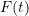as shown in Figure 7.9. This function can be considered to be composed of an infinite number of infinitesimal impulsive loads, a few of which are illustrated. Again, we are interested in the response of the mass at some arbitrary time. This response will be affected by all of the impulses that have been applied up to time. Consider one such impulse at time. The application of the impulse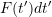atwill have an effect on the position of the mass for all later times, with the change in the position of the mass at timegiven by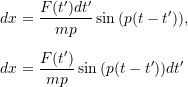exactly analogous to our previous results. As discussed previously, this actually represents the change in the response due to the application of this one impulse. To find the actual position of the mass at time, we simply add up (ie integrate) the effects of all the individual impulses up to time, which are the only ones that can affect the position at time. As a result, we get

(7.10)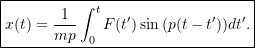(Note thatis simply a dummy variable.) Equation (7.10) is known as Duhamel’s Integral and is a special form of the convolution integral which gives the positionfor an arbitrary load. As discussed, this assumes that the initial conditions are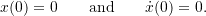If instead we have more general initial conditions given by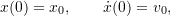then (7.10) must be replaced by the more general form

(7.11)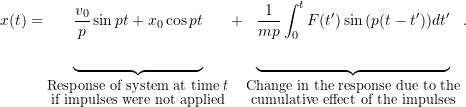#### Example 1

A mass, initially at rest, is subject to a step load as shown below. Determine the response of the systemfor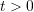. Use the method of convolution.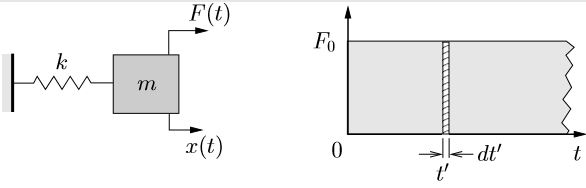#### Example 2

A mass, initially at rest, is subject to a ramp load as shown below. Determine the response of the systemfor. Use the method of convolution.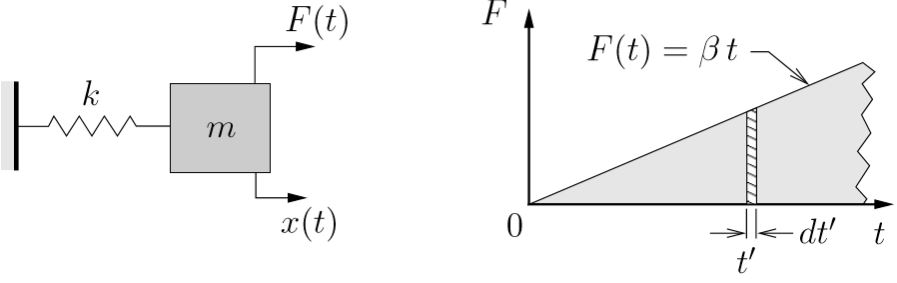#### Example 3

A mass, initially at rest, is subject to a rectangular step load as shown below. Using the the method of convolution, determine the response of the systemfor

1.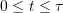,
2.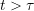.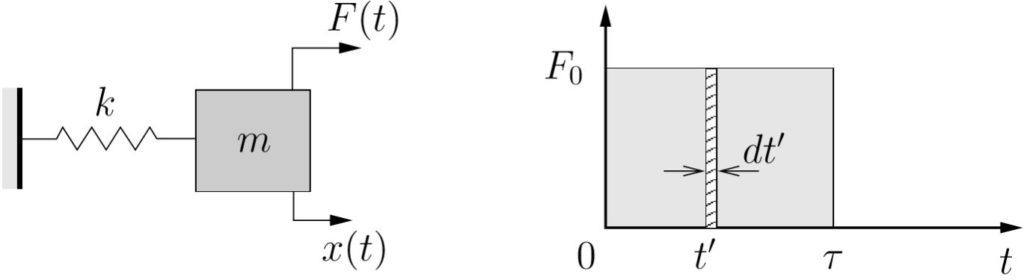#### Example 4

A mass, initially at rest, is subject to a forcing function shown below. Using the the method of convolution, determine the response of the systemfor

1.,
2..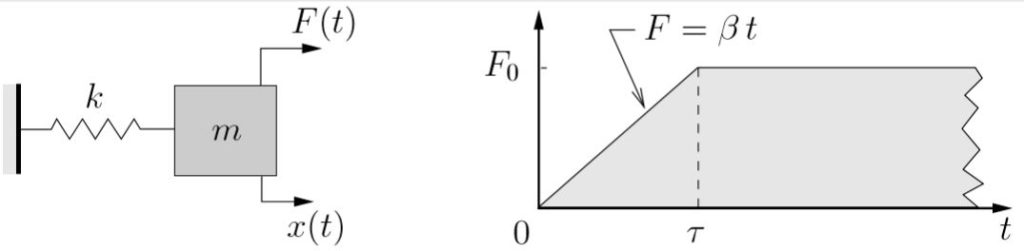#### Example 5

A mass is initially moving such that at some timethe mass has position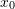and velocity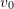. At that instant a step load is applied to the mass as shown below.
Determine the response of the systemforusing

1. Newton’s laws directly,
2. The method of convolution.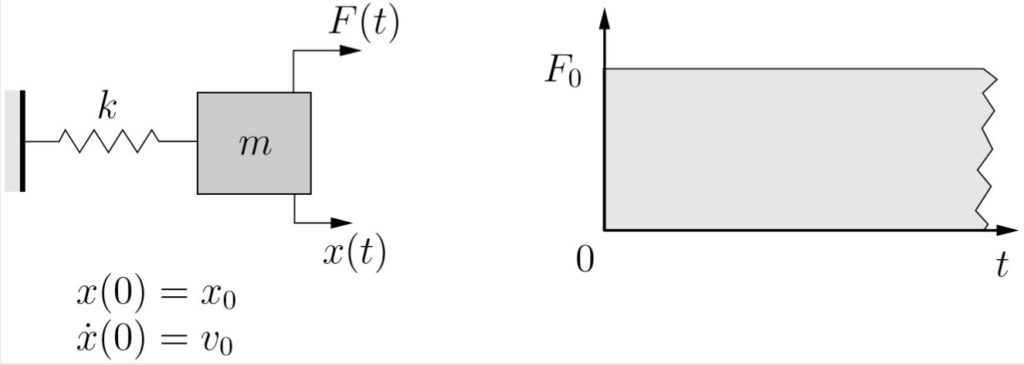### Convolution with Base Excitation

In some situations we do not apply a force directly to the mass but instead the base supporting the mass is given some motion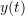. We have already seen that the equation of motion in such a case is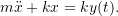This is identical to the equation of motion in equation (7.1) withreplaced by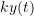. As a result, all of our previous results can be applied. In particular, Duhamel’s Integral (equation (7.10)) becomes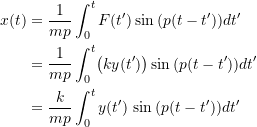or, since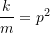,

(7.12)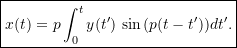### Convolution with Viscous Damping

So far we have only considered undamped situations. We will now consider the response of a spring–mass system with a viscous damper subjected to an impulsive load. We will again assume the initial conditions are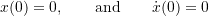before any impulses are applied. Similar to the undamped case, the initial conditions just after the impulse has been applied are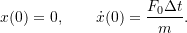The subsequent free vibration response is given by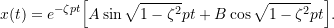With the given initial conditions, we find that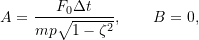so that the response becomes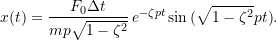If the impulse were instead applied at some later time, the response would be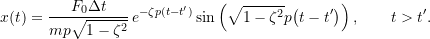Now as before we consider an arbitrary forcing function to be composed of an infinite number of infinitesimal impulses. The response at timedue to the impulse applied at some earlier timeis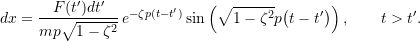The total response atis the cumulative effect of all the impulses applied up to time, ie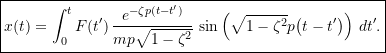If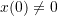or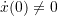before the impulses are applied this result needs to be generalized as before.